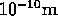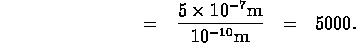PHYSICS 1020 Homework set 8
10 February 1997

[8.5]
If you doubled the wavelength of a wave without changing its wave speed, what would happen to the frequency? What if you halved the wavelength?
From the relation between speed, wavelength and frequency:we conclude that, with the wavespeed kept fixed, doubling the wavelength will halve the frequency, while halving the wavelength will double the frequency.

[8.8]
What happens to the energy of the two waves in Fig. 8.9 when they interfere destructively, as shown in the second of the three sketches? Did the energy vanish?
(Hint: what are the parts of the rope doing as the wave moves? Which type of energy is the wave carrying?
The wave is carrying kinetic energy corresponding to the up-and-down oscillatory motion of the elements of the rope. At the moment when the two opposite wave crests meet, the net displacement is zero, but the speed is not. The elements of the rope move through the zero displacement position at the instant when the displacements due to the two waves extinguish each other, but they still move. The energy does not disappear.

[8.12]
You shine two flashlights on the wall. Why don't you see an interference pattern?
The light emitted by flashlights is not a continuous stream of electromagnetic waves, but a sequence of rather short ``wavetrains'', each of which is independent of the others. Therefore they have no well-defined phase relation - they are not synchronized - they are ``incoherent''. Even though there is interference between these wavetrains, there is no point in space where they all interfere constructively or destructively. The resultant light intensity is the result of a random superposition of all these wave trains (or ``wave packets''), causing a rather uniform intensity distribution.

[8.18]
How does the operation of the electroscope (previous exercise) demonstrate electrical conduction?
In order for the charge deposited onto the electroscope to reach the leaves of the electroscope, charge must have moved from the charged rod (or whatever was used to deposit charges) through parts of the electroscope to the leaves. This motion (flow) of charges is called electrical conduction.

[8.26]
Making estimates: About how many atoms thick is a sheet of paper?
A 500-page stack (one package) of typing (or Xerox) paper is about 5 cm thick; so the thickness of one sheet is about 5 cm/500 = 0.1 mm =. The diameter of an atom is about, so the number of atoms across the thickness of one sheetSo, one sheet of paper is about 1 million atoms thick.

[8.27]
Making estimates: Which is bigger, an atom or a wavelength of light? Roughly how much bigger?
The diameter of an atom is about, while the wavelength of visible light (e.g. blue-green light) is about 500 nm =. The ratio between these two numbers